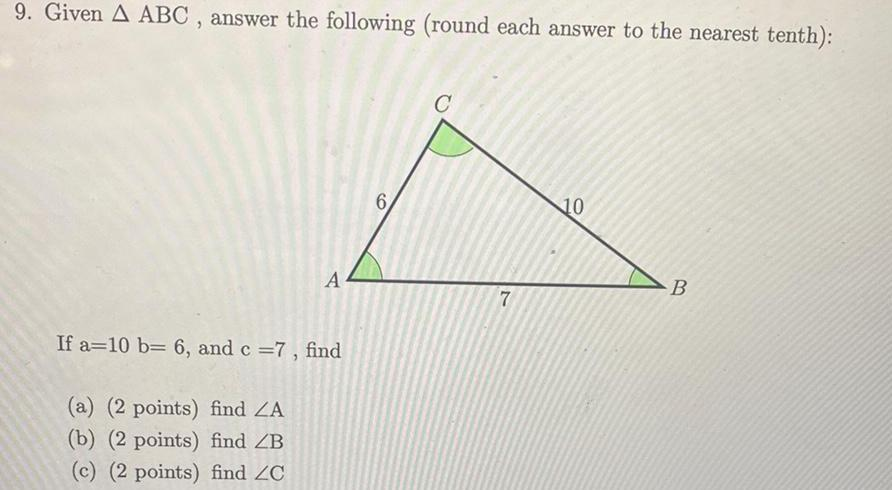Question:

# 9 Given A ABC answer the following round each answer to the

Last updated: 11/18/20239 Given A ABC answer the following round each answer to the nearest tenth A If a 10 b 6 and c 7 find a 2 points find ZA b 2 points find ZB c 2 points find ZC 6 C 7 10 B